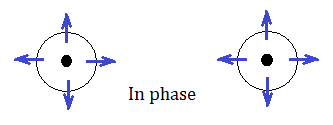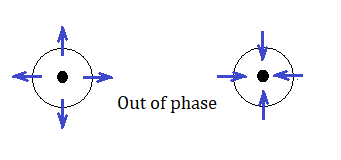# A physics question related to wave interference

vegbird
Homework Statement:
Straight line AB connects two point sources that are 5.00 m
apart, emit 300 Hz sound waves of the same amplitude, and emit
exactly out of phase. (a) What is the shortest distance between the
midpoint of AB and a point on AB where the interfering waves
cause maximum oscillation of the air molecules? What are the
(b) second and (c) third shortest distances?
Relevant Equations:
This is from my physics textbook, the solutions are a) 0; (b) 0.572 m; (c) 1.14 m
However, I do not understand it at all. Since two point sources at A and B are exactly out of phase. The mid point is a node where the amplitude is zero with zero oscillation. How can part (a) be zero? Should that be a quarter of the wavelength away from the mid point?

Homework Helper
Gold Member
2022 Award
I agree with you.

Edit.. which was a blunder.

Last edited:
••Homework Helper
Gold Member
I think the answers are correct.Here we have two point sources in phase. The two wavefronts shown have the same radius and denote points where the air molecules are at maximum displacement away from the source.Here the two point sources are out of phase. Air molecules for the wavefront of the left source are at maximum outward displacement while at the same time the air molecules for the corresponding wavefront of the right source are at maximum inward displacement.

When the two wavefronts of the out-of-phase sources meet in the middle, is there a displacement node or a displacement antinode?

••Roon and Delta2
Homework Helper
Gold Member
Hmm @TSny does it play a role here that sound is a longitudinal wave? If it was transverse wave?

Homework Helper
Gold Member
Hmm @TSny does it play a role here that sound is a longitudinal wave?
Yes, I believe so. But there could also be some ambiguity about the definition of “two point sources in phase”. For me, the two sources of longitudinal waves are in phase if they behave as I drew in the first diagram of my previous post. When one is pulsing radially outward, so is the other one.

But along the line connecting these two sources, when the left source is pulsing toward the right the other source is pulsing toward the left. So, some people might say that these two sources are in fact out of phase for waves propagating along the line connecting them. Someone who interpreted the wording “the two sources emit exactly out of phase” in this way would not agree with the answers given for the problem.

•Delta2
Homework Helper
The "trick" of the question is to realize the difference between the behaviour of the particle displacement and acoustic pressure in a sound wave in air. The longitudinal sound waves in a gas are usualy descrbed in terms of acosutic pressure. The maximum audible effect happens when the acoustic pressure is maximum. So here you do have a node in the middle but this means that the acoustic pressure is zero and you will detect no sound. However, the particle displacement follows an oscillation with 90o phase difference compared with the acoustic pressure. Maximum acoustic pressure means zero displacement and vice-versa.
Acoustic pressure (p) is the difference between the actual pressure and the equilibrium pressure. It can e positive or negative. In the middle point, one wave have positive p and the other negative p and the sum is zero. However, as @TSny has nicely showed in the diagram, the displacements are actually adding in the center and the result is a maximum displacement.